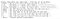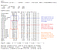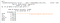# Diamonds are precious — and predictable!

We’re predicting diamonds today, care to join?

This is an inbuilt dataset in R-studio. We intend to predict the diamond prices based on the features available. There are 53940 records in the dataset. As a ritual, let’s split the data into a test (30%) and train (70%).

Now that we have our training data, let us check the structure of the dataset. I am using R for my analysis.What’s the deal with the 4Cs — Carat, Cut, Color, Clarity?

A fun part of being a data analyst is an opportunity to learn across domains, and today is no different. Before we analyze the data further, let us understand what each column in the dataset means. And who do we have to help us with that? Gemological Institute of America (GIA). So let’s decipher each of the 4Cs and other fields in the dataset.

1. Carat weight: Represents the weight of the diamond. Bigger, the better (if other factors are held constant).

2. Clarity: Refers to the absence of inclusions and blemishes. The clearer the diamond, the better the diamond, expensive the diamond (Other factors held constant).

3. Color: Color refers to the absence of color in diamonds.

4. Cut: Based on various dimensions, a diamond is assigned a cut rating or grade. This ranges from Excellent to Poor. Our dataset has the following cut ratings:

Fair < Good < Very Good < Premium < Ideal

Better the cut rating, expensive the diamond (other factors held constant).

5. Depth, table, x, y, z: These are physical dimensions of the diamond as below:

How do these relate to diamond prices? I have no idea, but hey — what’s data for! Let’s dive.

To begin with, let us check the distribution of our dependent variable.

Now that we know how the price varies, let us check its relation with independent variables (predictors) in the dataset.

Price v/s carat weight: As expected, an increase in carat weight would lead to an increase in diamond price.

Price v/s Cut type: One would expect the diamond prices to rise with a better cut, this does not seem to be the case in our dataset.

The diamond price should have been higher for a better cut grade, maybe there are other factors at play. The variation in carat weight is similar to that in the price, maybe carat weight is over-powering the cut grade? Maybe!

Price v/s color type: Based on our domain expertise, we should have seen price dropping from color type D to J. But here again, we are up for a surprise.

It seems that the carat weight is over-powering the color as shown below:

Price v/s clarity: The data does not align with our domain knowledge. We should have seen a price increase as the clarity improved, but that does not seem to be evident.

Upon inspection, carat seems to be influencing the price.

Depth v/s price: For a given depth, diamond prices fluctuate from high to low. Could be that there is no correlation between them.

Table v/s price: Association between table and price is also not noticeable because of the high variation in price across the range of table width.

x (length),y(width), and z(depth) v/s price and carat weight: Diamond prices tend to rise exponentially with an increase in dimensions.

The increase in dimensions such as x, y, or z also impacts the carat weight, therefore it makes sense to check for collinearity between these predictors. The correlation matrix is as shown below:

The relation between predictors is not linear, but monotonic. Hence Spearman’s correlation coefficient is considered instead of Pearson’s. Since x,y, and z are almost exactly collinear to carat weight and price, they will be dropped from the model here on.

One-hot encoding the categorical columns: Categorical variables such as clarity, color, and cut are represented with ordinal values. This, in my opinion, is incorrect since the difference between Fair and Good cut is not the same as Good and Very Good Cut. The same applies to other categorical fields as well. These columns are therefore one-hot encoded. The correlation matrix after one-hot encoding is as shown below:

One cannot help but notice the strong correlation between carat weight and price, perhaps we should start thinking about the baseline models hereon.

Choosing baseline models:

In the case of regression, very often we choose mean value as a baseline. Here, I will take both mean and median as the baseline model because the data is right-skewed and I believe the median will be a better choice.

We measure the efficiency of the models using the Mean Absolute Percentage Error (MAPE). The lower the MAPE, the better the model.

Baseline Mean has a MAPE of 188% on training data. Well, this is pathetic, but hey let’s fix it.

Baseline Median has a MAPE of 110% on training data. An improvement over Baseline Mean, but still a long way to go.

Think linear:

As we see from the correlation matrix above, Carat is perhaps a predictor with a strong association with diamond prices. We start our journey by fitting a linear model with carat weight as the predictor.

As expected, an increase in the carat weight increases the price of a diamond. The model built conforms to that notion. A negative intercept is obtained to minimize the sum of squared residuals. No significance to that can be attached, but a negative intercept would definitely underpredict the price for lower carat weights.

One must also be keen on knowing if the model’s predictive power is constant across the range of diamond prices?

The linear model with carat as the only predictor does not exhibit homoscedasticity.

The simple regression model has a MAPE of 38.3% on training data, a step in the right direction.

Let us now go ahead and include all the predictors and build a multiple regression.Predictors CutFair, ColorJ, and ClarityI1 are excluded from the model to prevent the dummy variable trap

All the predictors in the model are significant at a 1% significance level. An adjusted R-squared of 0.917 shows that the multiple linear regression model is good at explaining the variation in diamond price across its mean. Let us also check if the linear model would have stable prediction across the range of predicted values using residual v/s fitted plot.

The model continues to show inconsistent residuals across the range of predicted values. We must go beyond linear models and explore other options.

The multiple linear regression has a MAPE of 44.8% on training data. More the merrier? — Not here!

Decision trees: A wise man once said, if you are lazy (which I am) decision trees are for you. This is to say that if we are unaware/unsure of the actual relationship between our predictors and the response variables, try decision trees. Let us explore this option:

As noticed during EDA and regression models so far, carat weight seems to overpower other predictors in terms of determining the price value of a diamond. This is also evident from the splits. The model has a MAPE of 32.8% on training data, which isn’t impressive either.

Ensemble methods:

Decision trees often overfit the data. A way to prevent that from happening is to predict based on ensemble techniques where a number of trees are used to predict a value based on aggregation.

Bagging: We start our journey with Bagging. We create 10–30 bootstrap samples and build a decision tree across each one of them. We see that not out-of-bag error remains constant after 15 trees. Therefore a bagged model of 15 trees is chosen (Hyperparameter nbags= 15 after tuning, for geeks!).

This model has a MAPE of 32.3%, which again does not give us accurate predictions as expected.

Random Forest: We switch over to Random Forests where we build an ensemble of a number of trees (up to 150) and see that the out-of-bag error is almost constant after 100, but we still choose a Random Forest ensemble of 150 and aggregate our results.

We set the random forest to have 150 individual trees and predict the training model to see that it has a MAPE of 6.77%. Quite impressive!

Let us quickly glance over the residual v/s fitted plot if this impressive MAPE is actually true.

A mean residual of zero and a relatively stable prediction convinces us that we can trust the random forests built for our prediction. But hey, where is the interpretation?

This is where ensemble techniques such as bagging and random forest don’t do well. Since the output is a result of a number of trees aggregated, no single interpretation (as in a single decision tree) is available. These are also fondly called black-box models. What is easily accessible is the importance of each predictor in the model built, this is as shown below:150 trees in the random forest with carat as the most important feature for prediction

Let us now validate all our models on the test data (remember the 30% that we set aside in the beginning?). The results are as below:

The models perform consistently across train and test data.

Summary

We studied the 4Cs of Diamond and how they influence pricing.

Our baseline model had a MAPE of 188% on training data. Through a series of maneuvers (linear, multiple linear, decision tree, bagging), we were able to reduce the MAPE to 6.77% using Random Forest tree ensembles. The problem, however, is interpretability with Random Forests that led to lower MAPE (better model) but lacks interpretability.

Could there be a better model with a lower MAPE? Hell yeah! But for now, this is where I stop. I would keep the project and the article updated as and when there is something valuable to be added.

Source code to the project is available below: